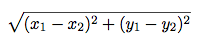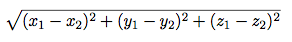If you are studying for an exam, or you just want some extra practice with concepts in this class, you might find these practice problems helpful!

### Question 1: Distance

Implement a function called `distance(x1, y1, x2, y2)`:

• `x1` and `y1` form an x-y coordinate pair
• `x2` and `y2` form an x-y coordinate pair

`distance` returns the Euclidean distance between the two points. Use the following formula:``````import sqrt

def distance(x1, y1, x2, y2):
"""Calculates the Euclidian distance between two points (x1, y1) and (x2, y2)

>>> distance(1, 1, 1, 2)
1.0
>>> distance(1, 3, 1, 1)
2.0
>>> distance(1, 2, 3, 4)
2.8284271247461903
"""
return sqrt(square(x1-x2) + square(y1-y2))``````

### Question 2: Distance (3-D)

Now, let us edit this program to get the distance between two 3-dimensional coordinates. Your `distance3d` function should take six arguments and compute the following:``````def distance3d(x1, y1, z1, x2, y2, z2):
"""Calculates the 3D Euclidian distance between two points (x1, y1, z1) and
(x2, y2, z2).

>>> distance3d(1, 1, 1, 1, 2, 1)
1.0
>>> distance3d(2, 3, 5, 5, 8, 3)
6.164414002968976
"""
return sqrt(square(x1-x2) + square(y1-y2) + square(z1-z2))``````

### Question 3

Implement `harmonic`, which returns the harmonic mean of two positive numbers `x` and `y`. The harmonic mean of 2 numbers is 2 divided by the sum of the reciprocals of the numbers. (The reciprocal of `x` is `1/x`.)

``````def harmonic(x, y):
"""Return the harmonic mean of x and y.

>>> harmonic(2, 6)
3.0
>>> harmonic(1, 1)
1.0
>>> harmonic(2.5, 7.5)
3.75

>>> harmonic(4, 12)
6.0
"""
return 2/(1/x + 1/y)``````

### Question 4: Environment diagrams

If you haven't found this gem already, tutor.composingprograms.com has a great visualization tool for environment diagrams. Paste in your Python code and it will generate an environment diagram you can walk through step-by-step! Use it to help you check your answers!

Try drawing environment diagrams for the following examples and predicting what Python will output:

``````>>> def square(x):
...     return x * x
>>> def double(x):
...     return x + x
>>> a = square(double(4))
>>> a
______64``````
``````>>> x, y = 4, 3
>>> def reassign(arg1, arg2):
...     x = arg1
...     y = arg2
>>> reassign(5, 6)
>>> x
______4
>>> y
______3``````
``````>>> def f(x):
...     f(x)
>>> print, f = f, print
>>> a = f(4)
______4
>>> a
______# Nothing shows up, because a = None
>>> b = print(4)
______4
>>> b
______# Nothing shows up, because b = None``````